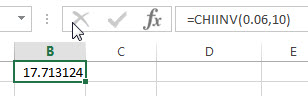## Excel CHIINV function

This post will guide you how to use the CHIINV function with syntax and examples in Microsoft excel.

### Description

The Excel CHIINV function returns the inverse of the right-tailed probability of the chi-squared distribution.  For example, if the probability is equal to CHIDIST(x,…), then the CHIINV(probability,…) is equal to x.

The CHIINV function is a build-in function in Microsoft Excel and it is categorized as a Statistical Function.

The CHIINV function is available in Excel 2016, Excel 2013, Excel 2010, Excel 2007, Excel 2003, Excel XP, Excel 2000, Excel 2011 for Mac.

### Syntax

The syntax of the CHIINV function is as below:

`= CHIINV (probability, deg_freedom)`

Where the CHIINV function arguments are:

• Probability -This is a required argument. The probability for the chi-squared distribution.
• deg_freedom – This is a required argument.  The number of degrees of freedom. (the value must be a positive integer)

Note:

• If any argument is non-numeric, the CHIINV function will return #VALUE! Error.
• If deg_freedom argument is not integer and the CHIINV function will truncate it.
• If deg_freedom argument is smaller than 1, the CHIINV function will return the #NUM! Error.
• If probability is smaller than 0 or greater than 1, then the CHIINV function will return the #NUM! Error.

### Excel CHIINV Function Examples

The below examples will show you how to use Excel CHIINV Function to get the inverse of the right-tailed probability of the chi-squared distribution.

1# to calculate the right-tailed probability of the chi-square distribution, using the following formula:

=CHIINV(0.06,10)### Related Functions

• Excel CHIDIST function
The Excel CHIDIST function returns the right-tailed probability of the chi-squared distribution.The syntax of the CHIDIST function is as below:= CHIDIST (x,deg_freedom).…
• Excel CHITEST Function
The Excel CHITEST function returns the value from the chi-squared distribution for the statistic and the appropriate degrees of freedom. The syntax of the CHITEST function is as below:= CHITEST(actual_range, expected_range)…